# Test: Maxima And Minima (Competition Level) - 1

## 30 Questions MCQ Test Mathematics (Maths) Class 12 | Test: Maxima And Minima (Competition Level) - 1

Description
This mock test of Test: Maxima And Minima (Competition Level) - 1 for JEE helps you for every JEE entrance exam. This contains 30 Multiple Choice Questions for JEE Test: Maxima And Minima (Competition Level) - 1 (mcq) to study with solutions a complete question bank. The solved questions answers in this Test: Maxima And Minima (Competition Level) - 1 quiz give you a good mix of easy questions and tough questions. JEE students definitely take this Test: Maxima And Minima (Competition Level) - 1 exercise for a better result in the exam. You can find other Test: Maxima And Minima (Competition Level) - 1 extra questions, long questions & short questions for JEE on EduRev as well by searching above.
QUESTION: 1

### f(x) = 1 + 2x2 + 4x4 + 6x6 + ................+ 100x100 is polynomial in a real variable x, then f(x) has

Solution:

f(x) = 1 + 2x2 + 4x4 +...............+ 100x100
= f’(x) = 4x + (4)2 x3 + (6)2 x5 +......................+ (100)2 x99
f’(x) = x[(2)2 + (4)2 x2 + (6)2 x4 +....................+ (100)2 x98
f’(x) = 0(only one minimum)

QUESTION: 2

### On the interval [0, 1] the function x25(1 – x)75 takes its maximum value at

Solution:

Let f(x)=x25(1−x)75,xϵ[0,1]
⇒f′(x)=25x24(1−x)75−75x25(1−x)74
=25x24(1−x)74{(1−x)−3x}
=25x24(1−x)74(1−4x)
We can see that f′(x) is positive for x<1/4 and f'(x) is negative for x>1/4
Hence, f(x) attains maximum at x= 1/4

QUESTION: 3

### The product of minimum value of xx and maximum value of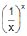is

Solution:

f(x) = xx     f(x) = x(-x)
f’(x) = xx(1+ln x)          f’(x) = -x(-x)(1+ln x)
1+ln x = 0                   x = 1/e
x = 1/e
Min value = (1/e)(1/e)        f(1/e) = e(1/e)
Product = (e-1/e)(e)1/e = 1

QUESTION: 4

The minimum value of the function defined by f(x) = max (x, x + 1, 2 – x) is

Solution:

f(x) = max(x, x+1, 2-x)
y = 2-x
y = x+1
Comparing both the equations, we get
2 - x = x + 1
x = ½
y = 2 - x
y = 2 - (½)
y = 1/3

QUESTION: 5

Let f(x) =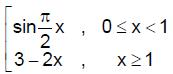then

Solution:

f(x) has local maxima at x = 1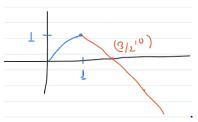QUESTION: 6

The greatest and the least values of the function, f(x) = 2 –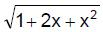, x ∈ [–2, 1] are

Solution:
QUESTION: 7

Let f(x) = {x}, For f(x), x = 5 is (where {*} denotes the fractional part)

Solution:
QUESTION: 8

The critical points of f(x) =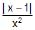lies at

Solution:
QUESTION: 9

The difference between the greatest and least values of the function f(x) = sin 2x – x on [–p/2, p/2] is

Solution:
QUESTION: 10

The radius of a right circular cylinder of greatest curved surface which can be inscribed in a given right circular cone is

Solution:

OE = x, OC = r, AO = h
∠AOC ≅ ∠QEC
AO / OC = QE/EC
(QE) = AO/OC(EC)
=h / r (r − x)
δx = 2πx (h / r(r − x))
=2 πhr (rx − x2)
δ'(x) = 0
x = r/2
derivating again
`delta''(r/2) = (2pih) / r(-2) < 0
r/2 is at maxima

QUESTION: 11

The dimensions of the rectangle of maximum area that can be inscribed in the ellipse (x/4)2 + (y/3)2 = 1 are

Solution:
QUESTION: 12

The largest area of a rectangle which has one side on the x–axis and the two vertices on the curve y =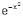is

Solution:
QUESTION: 13

The co–ordinates of the point on the curve x2 = 4y, which is at least distance from the line

y = x – 4 is

Solution:
QUESTION: 14

f(x) =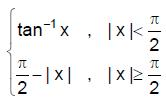then

Solution:
QUESTION: 15

Let f(x) =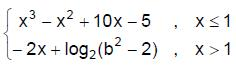the set of values of b for which f(x) has greatest value at x = 1 is given by

Solution:
QUESTION: 16

The set of values of p for which the extrema of the function, f(x) = x3 – 3px2 + 3(p2 – 1) x + 1 lie in the interval (–2, 4) is

Solution:

f(x)=x3−3px2+3(p2−1)x+1
f′(x)=3{x2−2px+(p−1)(p+1)}
f′(x)=3{x−(p−1)}{x−(p+1)}
Using given condition,
−2<p−1<4 and −2<p+1<4
⇒−1<p<5 and −3<p<3
∴p∈(−1,3)

QUESTION: 17

Four points A, B, C, D lie in that order on the parabola y = ax2 + bx + c. The co–ordinates of A, B & D are known as A(–2, 3); B(–1, 1) and D(2, 7). The co–ordinates of C for which the area of the quadrilateral ABCD is greatest is

Solution:
QUESTION: 18

In a regular triangular prism the distance from the centre of one base to one of the vertices of the other base is l. The altitude of the prism for which the volume is greatest is

Solution:
QUESTION: 19

Two vertices of a rectangle are on the positive x–axis. The other two vertices lie on the lines y = 4x and y = –5x + 6. Then the maximum area of the rectangle is

Solution:

If the base of the rectangle is the segment (a,0) to (b,0) then the upper vertices are (a,4a) and (b,6-5b).
Since it is a rectangle, the height is the same, so 4a = 6-5b.
That is, b = (6-4a)/5
Now, the area is (b-a)(4a) = ((6-4a)/5-a)(4a) = 12a/5 (2-3a)
This is a parabola with vertex at (1/3, 4/5).
check: So, b=14/15 and the maximum area is
(14/15 - 1/3)(4/3) = (9/15)(4/3) = 4/5

QUESTION: 20

A variable point P is chosen on the straight line x + y = 4 and tangents PA and PB are drawn from it to circle x2 + y2 = 1. Then the position of P for the smallest length of chord of contact AB is

Solution:
QUESTION: 21

The maximum area of the rectangle whose sides pass through the angular points of a given rectangle of sides a and b is

Solution: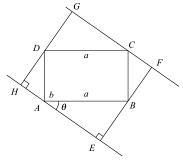A = Area EFGH = (bsinθ + acosθ) (acosθ + bcosθ)
= ab(a2 + b2)sinθcosθ
d2A/dθ2 = 1-(2)(a2 + b2)sin2θ,
so d2A/dθ2|<0    {θ = pi/4}
Hence A(max) = ½(a+b)2

QUESTION: 22

If p and q are positive real numbers such that p2 + q2 = 1, then the maximum value of (p + q) is

Solution:
QUESTION: 23

The function f(x) =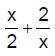has a local minimum at

Solution:

f(x)=x/2 + 2/x
f`(x)=1/2-2/x²
For extremum f ′(x)=0
>0 for x=2
⇒ 1/2-2/x²=0
⇒ x=±2
f′′(x)=4/x³>0 for x=2
∴ x=2 is the point of minima
∴ Given function has local minima at x=2.

QUESTION: 24

If x is real, the maximum value of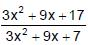is

Solution:
QUESTION: 25

If the function f(x) = 2x3 – 9ax2 + 12a2x + 1, where a > 0, attains its maximum and minimum at p and q respectively such that p2 = q, then a equals

Solution:
QUESTION: 26

The maximum value x3 – 3x in the interval [0, 2] is

Solution:
QUESTION: 27

Minimum value of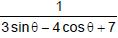is

Solution:
QUESTION: 28

The minimum value of (x – p)2 + (x – q)2 + (x – r)2 will be at x equals to

Solution:
QUESTION: 29

The number of values of x where f(x) = cos x + cos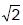x attains its maximum value is

Solution:

f′(x) =−sinx− (2)1/2sin((2)1/2x)
Hence x = 0
Hence maximum value will be attained at x=0
f(0)=2
The maximum value of f(x) = cos x + cos (√2x) is 2 which occurs at x = 0. Also, there is no other value of x for which this value will be attained again.

QUESTION: 30

The maximum value of cos a1 . cos a2 . cos a3....cos an under the restriction 0 £ a1, a2,.....an £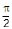and cot a1 cot a.... cot an=1 is

Solution:

Given condition cotα1.cotα2.............cotαn=1
⇒cosα1.cosα2.......cosαn
​=sinα1.sinα2.........sinαn
​This is possible only when  α1=α2=α3=....=αn
​=45o
⇒cosα1.cosα2.......cosαn = 1/(2)1/2 . 1/(2)1/2 ......... 1/(2)1/2 =1/ (2)n/2